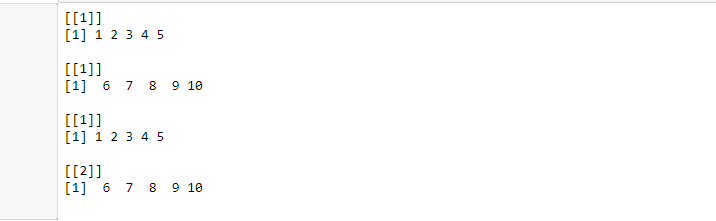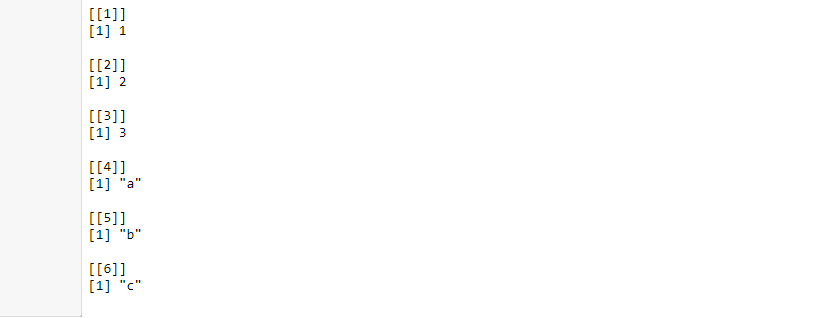GeeksforGeeks App
Open AppBrowser
Continue

# How to combine two lists in R?

In this article, we are going to combine two lists in R Language using inbuilt functions. R provided two inbuilt functions named c() and append() to combine two or more lists.

Method 1: Using c() function

c() function in R language accepts two or more lists as parameters and returns another list with the elements of both the lists.

Syntax:

c(list1, list2)

Example 1:

## R

 `# R Program to combine two lists`` ` `# Creating Lists using the list() function``List1 <- ``list``(1:5)``List2 <- ``list``(6:10)`` ` `print``(List1)``print``(List2)`` ` `# Combining lists using c() function``List3 = ``c``(List1, List2)``print``(List3)`

Output:Here, you can see that the second list has 2 elements, which shows that there are two lists combined as one.

Example 2:

## R

 `# R Program to combine two lists`` ` `# Creating Lists using the list() function``List1 <- ``list``(1, 2, 3)``List2 <- ``list``(``'a'``, ``'b'``, ``'c'``)`` ` `# Combining lists using c() function``List3 = ``c``(List1, List2)``print``(List3)`

Output:Method 2: Using append() function

append() function in R language accepts two or more lists as parameters and returns another list with the elements of both the lists.

Syntax:

append(list1, list2)

Example 1:

## R

 `# R Program to combine two lists`` ` `# Creating Lists using the list() function``List1 <- ``list``(1:5)``List2 <- ``list``(6:10)`` ` `print``(List1)``print``(List2)`` ` `# Combining lists using append() function``List3 = ``append``(List1, List2)``print``(List3)`

Output:Example 2:

## R

 `# R Program to combine two lists`` ` `# Creating Lists using the list() function``List1 <- ``list``(1, 2, 3)``List2 <- ``list``(``'a'``, ``'b'``, ``'c'``)`` ` `# Combining lists using append() function``List3 = ``append``(List1, List2)``print``(List3)`

Output:My Personal Notes arrow_drop_up